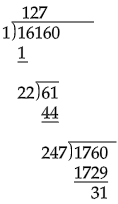# Find the least numbers which must be subtracted from the following number make them perfect squares: $16160$.

Given: A number $16160$.

To do: to find the least number to be subtracted from the number to make it perfect square.

Solution:

By using the long division method:-Here we find that $16160$ is $31$ more than $127^2$.

Thus, $31$ should be subtracted from $16160$ to make it perfect square.